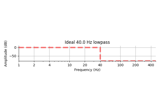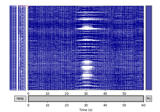# mne.viz.plot_filter¶

mne.viz.plot_filter(h, sfreq, freq=None, gain=None, title=None, color='#1f77b4', flim=None, fscale='log', alim=(- 80, 10), show=True, compensate=False, plot=('time', 'magnitude', 'delay'), axes=None)[source]

Plot properties of a filter.

Parameters
h

An IIR dict or 1D ndarray of coefficients (for FIR filter).

sfreq`float`

Sample rate of the data (Hz).

freq

The ideal response frequencies to plot (must be in ascending order). If None (default), do not plot the ideal response.

gain

The ideal response gains to plot. If None (default), do not plot the ideal response.

title

The title to use. If None (default), determine the title based on the type of the system.

colorcolor object

The color to use (default ‘#1f77b4’).

flim

If not None, the x-axis frequency limits (Hz) to use. If None, freq will be used. If None (default) and freq is None, `(0.1, sfreq / 2.)` will be used.

fscale`str`

Frequency scaling to use, can be “log” (default) or “linear”.

alim`tuple`

The y-axis amplitude limits (dB) to use (default: (-60, 10)).

showbool

Show figure if True (default).

compensatebool

If True, compensate for the filter delay (phase will not be shown).

• For linear-phase FIR filters, this visualizes the filter coefficients assuming that the output will be shifted by `N // 2`.

• For IIR filters, this changes the filter coefficient display by filtering backward and forward, and the frequency response by squaring it.

New in version 0.18.

plot

A list of the requested plots from `time`, `magnitude` and `delay`. Default is to plot all three filter properties (‘time’, ‘magnitude’, ‘delay’).

New in version 0.21.0.

axesinstance of `Axes` | `list` | `None`

The axes to plot to. If list, the list must be a list of Axes of the same length as the number of requested plot types. If instance of Axes, there must be only one filter property plotted. Defaults to `None`.

New in version 0.21.0.

Returns
fig`matplotlib.figure.Figure`

The figure containing the plots.

Notes

New in version 0.14.

## Examples using `mne.viz.plot_filter`¶